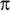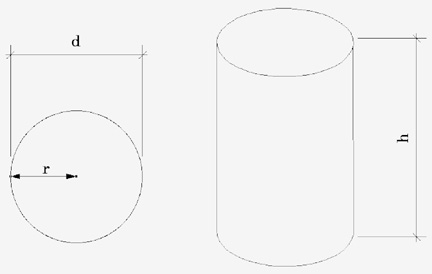Cubic Capacity

Cubic Capacity

The size of an engine is referred to as it's cubic capacity and is pretty easy to work out. Basically you are trying to calculate the area of a cylinder that is from the top of the piston at bottom dead center, to the top of the barrel. The red cylinder shows the area that cubic capacity calculates in the animation below.Skip straight to the cc calculator

To determine the volume of a cylinder you use the following formula:= 3.14 which is a fixed figure - you can calculate it to more decimals if you want to.

r = radius of the circle (in this case 1/2 the bore dimension or 'd' in the diagram below) in centimeters (ex: if the bore is 47mm that's 4.7 cm).

h = the height of the cylinder (in this case the stroke of the crank) in centimeters (ex: if the stroke is 51mm then it is 5.1cm).Below is a handy calculator sent to me by a scooterhelp.com user which will work out any cubic capacity to 2 decimal places

Bore (in mm)
Stroke (in mm)